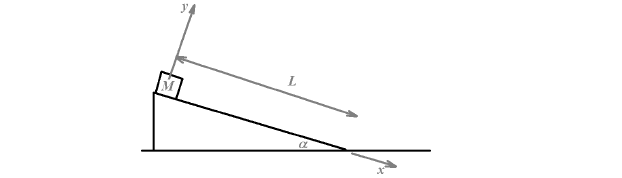# Solution: A mass M is initially held at rest at the top of an incline of length L, with slope forming an angle α with the horizontal (see figure). When the mass is released, it starts sliding down the incline. The coefficient of kinetic friction between the mass and the incline is μk.a) Draw a free body diagram for the mass.b) Find the acceleration of the mass.c) Find the time it take the mass to reach the bottom of the incline.Write your results in terms of M, L, α, μk, and g. Check the units/dimensions for each answer.

###### Problem

A mass M is initially held at rest at the top of an incline of length L, with slope forming an angle α with the horizontal (see figure). When the mass is released, it starts sliding down the incline. The coefficient of kinetic friction between the mass and the incline is μk.
a) Draw a free body diagram for the mass.
b) Find the acceleration of the mass.
c) Find the time it take the mass to reach the bottom of the incline.
Write your results in terms of M, L, α, μk, and g. Check the units/dimensions for each answer.